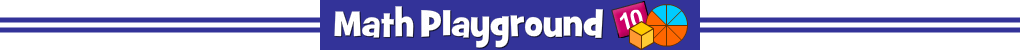Choose the correct fraction or decimal to win the race.
Look carefully at the comparison symbol.
Does the symbol mean less than, greater than, or equal to?
Choose a fraction or decimal on the board that will make each math statement true.
Monster Stroll Fractions - Learning Connections
Essential Skills
Mental Math - simplify and compare fractions

Common Core Connection for Grades 3 and 4
Explain equivalence of fractions and compare fractions by reasoning about their size.
Recognize and generate simple equivalent fractions.
Use comparison symbols when reasoning about fraction size.
Compare two fractions with different numerators and different denominators.
More Math Games to Play